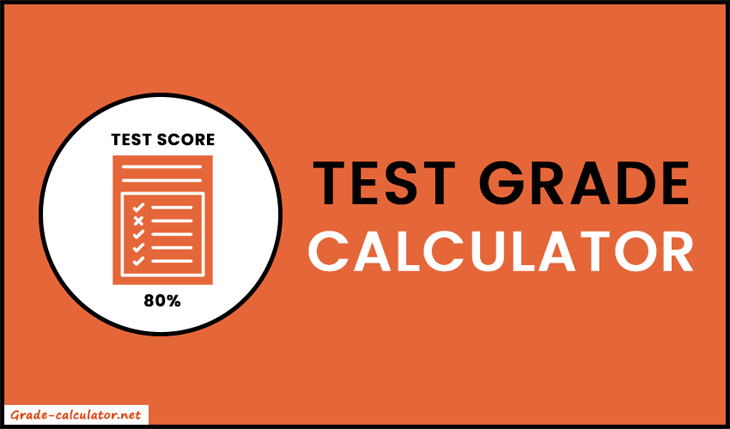Test Grade Calculator or Easy Grader lets you find percentage and letter grades on the basis of a number of questions and wrong answers.

%
No. of
Questions
No. of
(letter)

With Test Grade Calculator, you can easily determine your grade percentage and letter grade based on the total number of questions and the number of wrong answers. It's also known as a Test Score Calculator or Easy Grader. This can be useful for students, teachers, and anyone else who wants to quickly and accurately calculate test grades.

Simply adjust the values and get quick results on your end. Also, our tool shows the grade table including grade scores (in percentage and letter) with all possible wrong answers.### How to Calculate Test Score?

Percentage grade = 100 × ((Total Questions - Wrong Answers) / Total Questions)

Here's an example:

Suppose a test has 60 questions. A student got 6 wrong answers on the test. Find out the percentage and letter grade achieved by the student.

Now place all the given values into the formula.

Percentage grade = 100 × ((60 - 6) / 60)

= 100 × (54/60)

= 100 × 0.9

= 90%

So, the student got 90% on the test. Now check the letter grade for 90% in the grade table and we get A or A-.

### How to use Test Grade Calculator?

1. Firstly, select the grade scale in which you want to find your letter grade. Our test grade calculator shows both scales A, B, C, ... or A+, A-, B+, B-, ...
2. Now enter the total number of questions and wrong answers in the appropriate input fields.
3. Also, you can use the "+ Wrong" button to add up more wrong answers.
4. After entering all required values, just sit back and relax. Let our calculator do the rest for you.
5. As a result, it shows the percentage and letter grade on your screen. In addition, it shows the fraction grade achieved by you.
6. Use the "Reset" button for re-calculation.